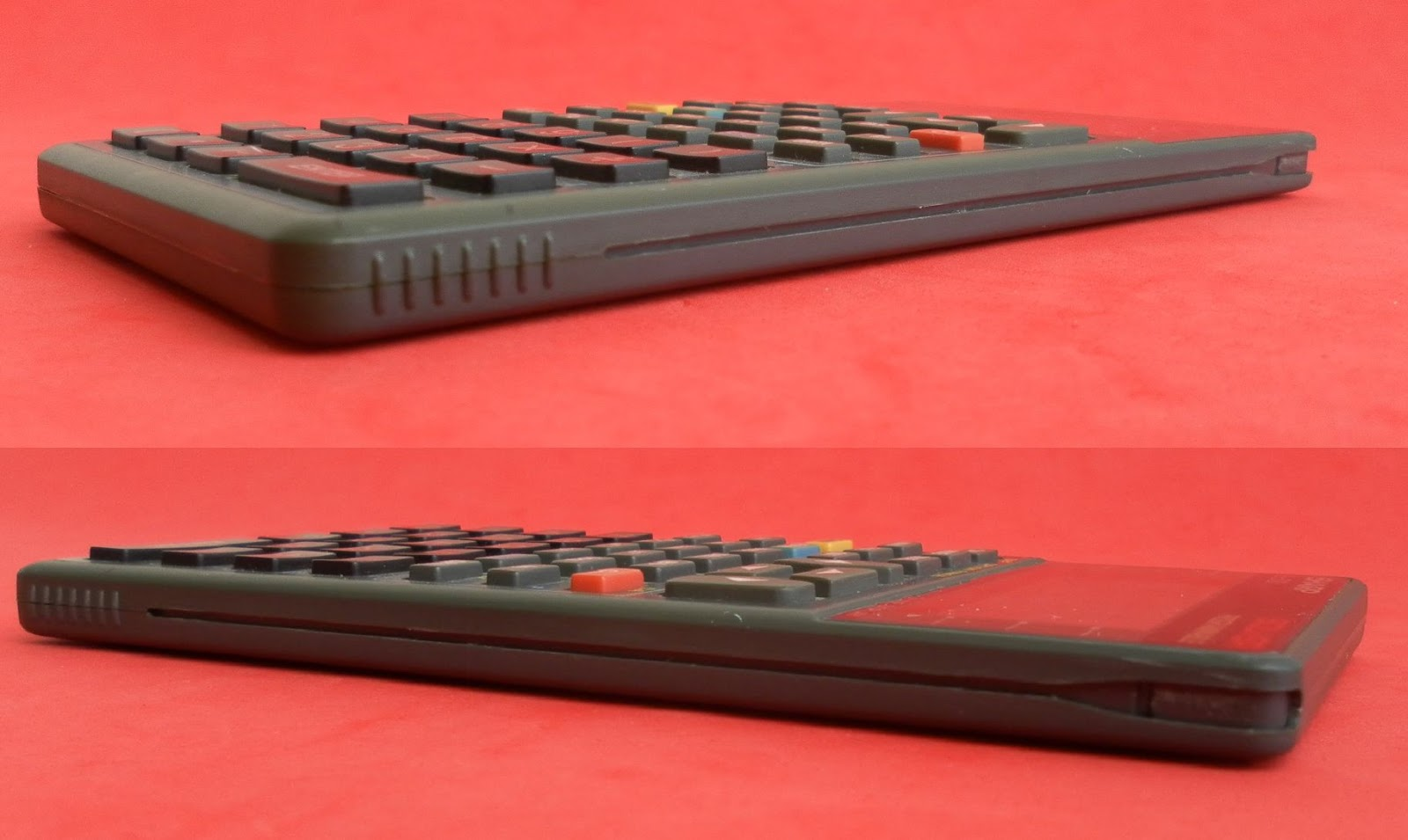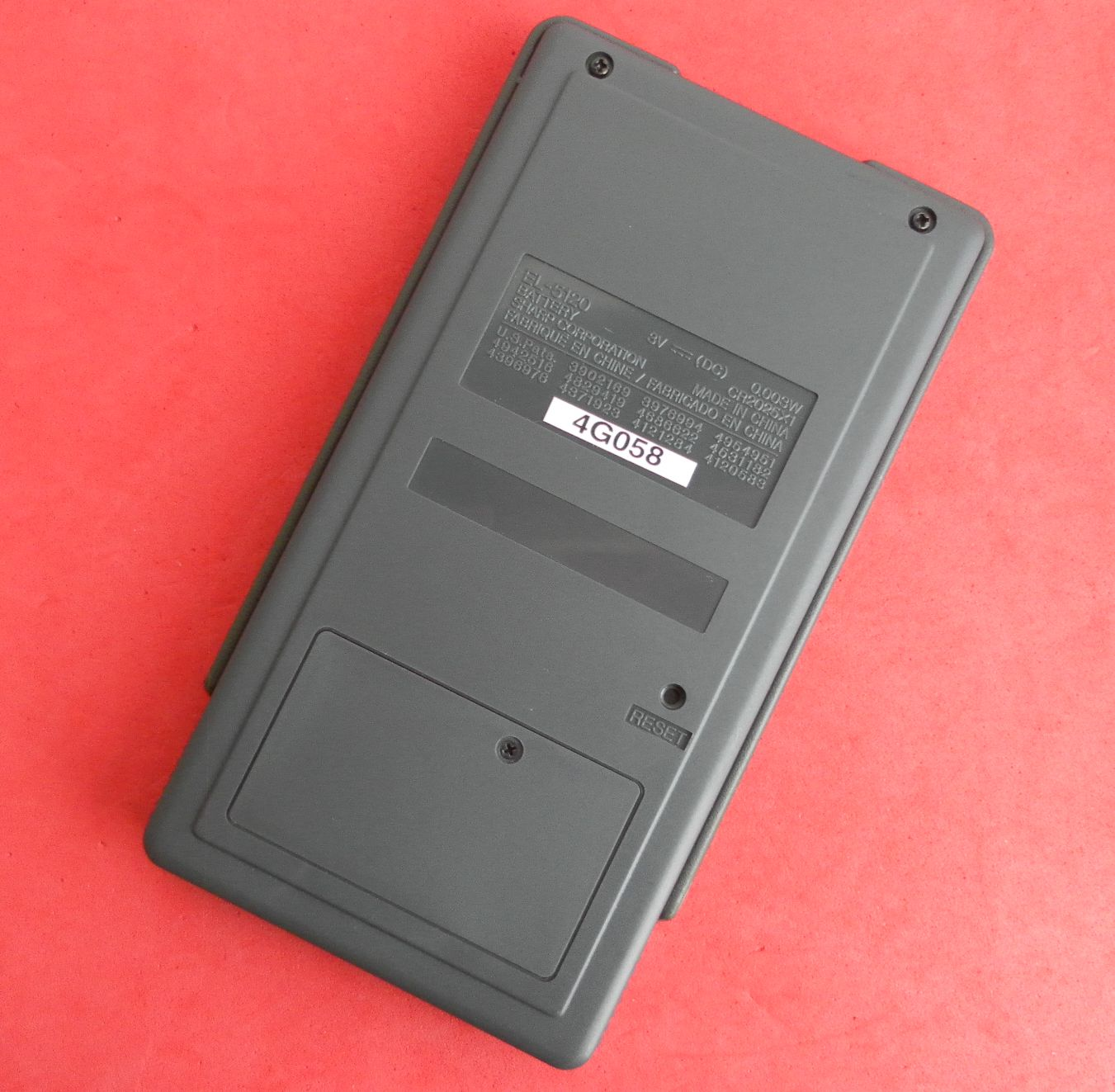SHARP EL-5120 Programmable Scientific Calculator: SOLVER machine with ENTER key?
03-05-2017, 03:01 PM (This post was last modified: 03-05-2017 03:15 PM by jebem.)
Post: #1jebemSenior Member Posts: 1,311 Joined: Feb 2014
SHARP EL-5120 Programmable Scientific Calculator: SOLVER machine with ENTER key?
Compact, well built, direct algebraic logic calculator, with a handy uncommon 3 lines alphanumeric characters with very high contrast black LCD display, from 1994.

Obviously the SOLVER capacity is the main feature of the model complemented by its programming ability. I didn't spend my time checking it in detail, but here are some highlights taken from the manual:

Powered by a single CR-2025 battery cell. 3mW power consumption at 3VDC.
Contrast adjust by software.

Display: 14 characters and 2 exponents x 3 rows, 5x5 dot matrix.
Number of display digits: 10 mantissa + 2 exponent
Number of internal calculation digits: 12 mantissa

Variables:
• 27 named global variables (A,..,Z, θ) persistent between operation modes.
• 9 additional named local variables in each equation or program.
• Last Answer memory.
Total user memory capacity: 1211 Byte.
Memory manager shows free memory.
Memory is shared between Variables, Programs and Equations.
Each variable consumes 8Byte (where its name takes 1Byte).
Each new Program structure takes 32 Byte; each line takes 3Byte plus the number of characters (1Byte each) or commands (1Byte each).

Setup allows to chose Deg, Rad, Grad, Floating, Fixed, Scientific, Engineering, Fractions.

It has four Operation Modes:
• REAL - Using real numbers, perform standard operations, expression solver calculations, integration and statistical operations on one & two variable, factorials, combinations and permutations, as well as polar-rectangular;
• NBASE - Perform binary, octal, decimal and hexadecimal operations and conversions;
• SOLV - Calculate unknown variables using an equation;
• PROG - Create and use named programs in REAL or NBASE modes to automate simple or complex calculations. BASIC alike programming with input, print, wait, rem, end, labels, goto, gosub, return, if/then, logical conditions, and statistical commands.

It supports three ways to calculate an unknown variable concerning its SOLVER calculation methods:
• Expression solver - Uses substitution;
• Integration - Uses Simpson rule;
• Solver functions - Uses Newton rule.Packaging and documentation.Forensic test result: 8.99998762105Compact pocket calculator.Jose Mesquita